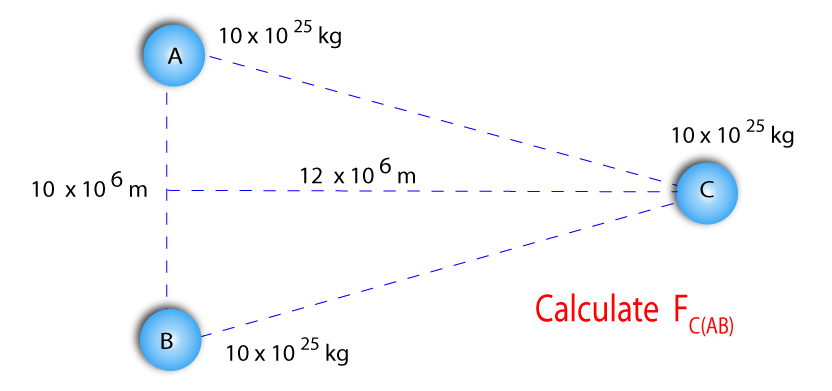## Learning Outcomes

Aids to Understanding

Homework Due Friday

## Suggested Homework..Try this for Friday 14th October

Suppose we wish to put a spacecraft in a geo-stationary orbit around the Earth. The Earth's mass is 6.0 x 1024 kg, the Earth's radius is 6.3 x 106 m and the height of a geo-stationary orbit is 3.6 x 107 m.
1. (it might be a good idea to draw the situation roughly to scale)

i) Calculate the gravitational field strength at this point.
ii) Calculate the force on the spacecraft, of mass 1000 kg, at this point.
iii) Calculate the gravitational potential at this point.
iv) Calculate the gravitational potential energy of a spacecraft at this point.
v) Calculate the speed of the spacecraft at this point.
vi) Calculate the kinetic energy of the spacecraft at this point.
vii) Calculate the total energy of the spacecraft at this point.
viii) By what factor is the answer to vi) larger than v)?
ix) Suppose the spacecraft wishes to leave the Earth and travel to the outer reaches of the solar system to take some pictures. What minimum speed will the spacecraft need to reach?